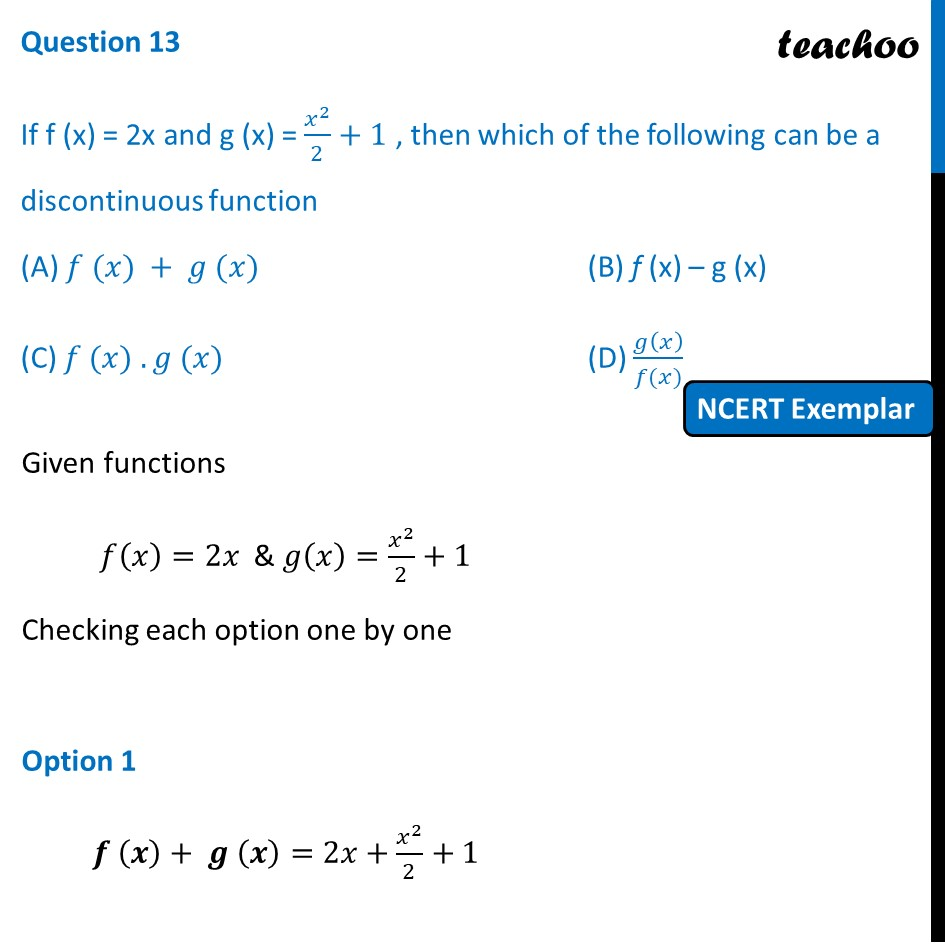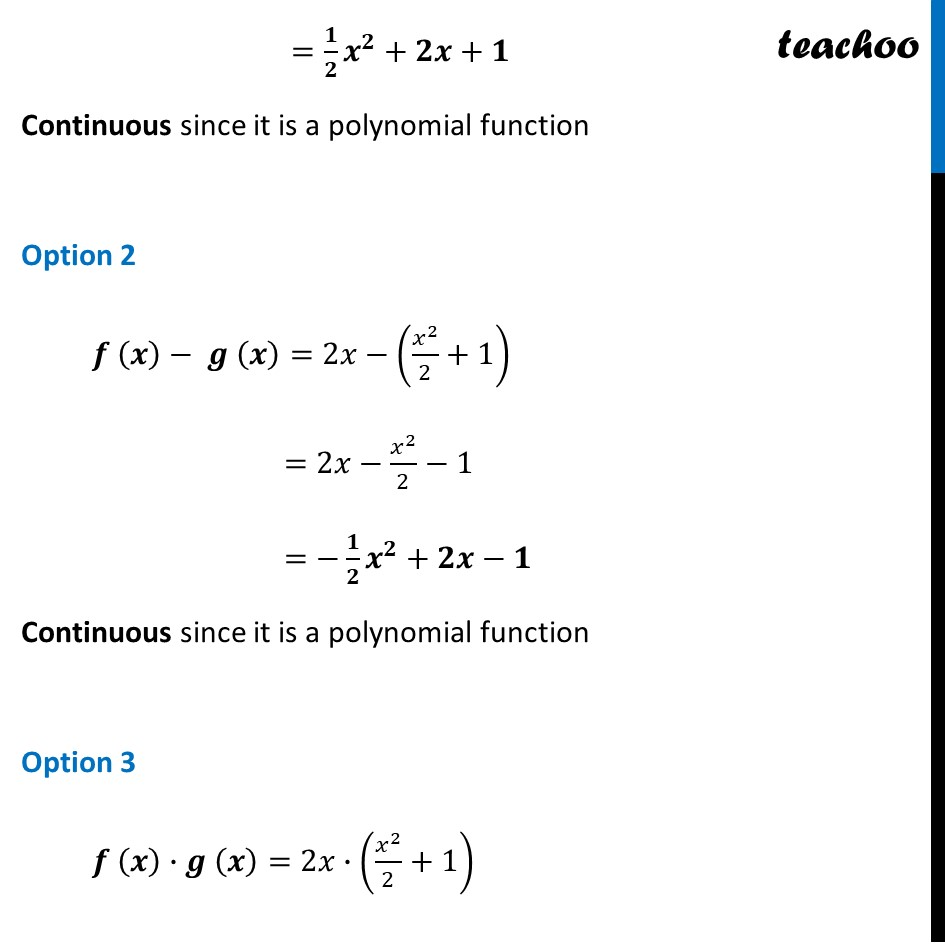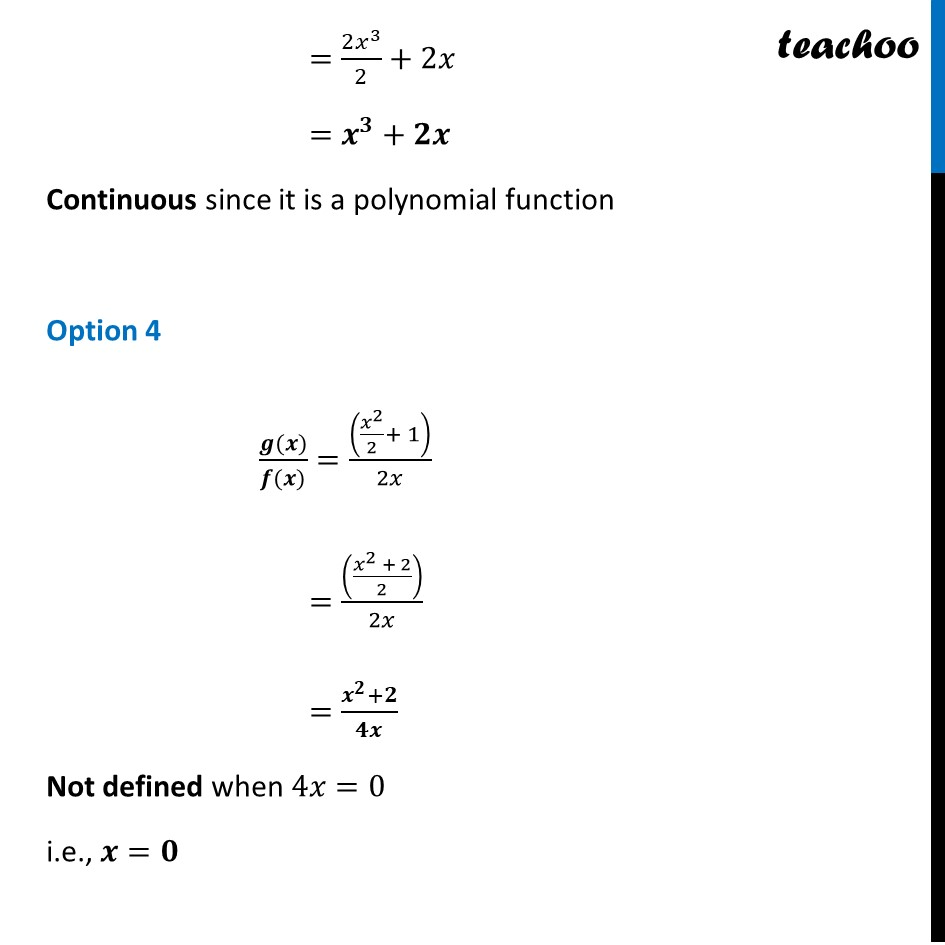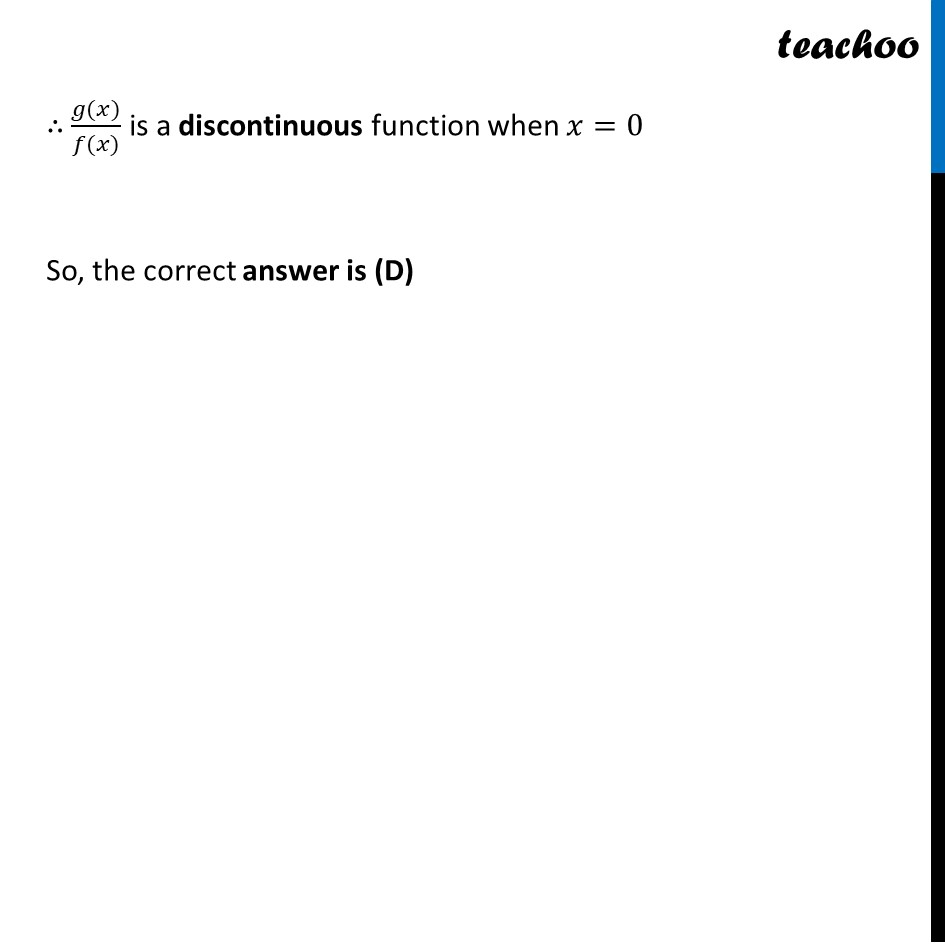NCERT Exemplar - MCQs

Chapter 5 Class 12 Continuity and Differentiability
Serial order wise

## (D) g(x)/(f(x))

This question is similar to Ex 5.1, 21 - Chapter 5 Class 12 - Continuity and DifferentiabilityLearn in your speed, with individual attention - Teachoo Maths 1-on-1 Class

### Transcript

Question 11 If f (x) = 2x and g (x) = 𝑥^2/2+1 , then which of the following can be a discontinuous function (A) 𝑓 (𝑥) + 𝑔 (𝑥) (B) f (x) – g (x) (C) 𝑓 (𝑥) . 𝑔 (𝑥) (D) 𝑔(𝑥)/(𝑓(𝑥)) Given functions 𝑓(𝑥)=2𝑥 & 𝑔(𝑥)=𝑥^2/2+1 Checking each option one by one Option 1 𝒇 (𝒙)+ 𝒈 (𝒙)=2𝑥+𝑥^2/2+1 =𝟏/𝟐 𝒙^𝟐+𝟐𝒙+𝟏 Continuous since it is a polynomial function Option 2 𝒇 (𝒙)− 𝒈 (𝒙)=2𝑥−(𝑥^2/2+1) =2𝑥−𝑥^2/2−1 =−𝟏/𝟐 𝒙^𝟐+𝟐𝒙−𝟏 Continuous since it is a polynomial function Option 3 𝒇 (𝒙)∙𝒈 (𝒙)=2𝑥∙(𝑥^2/2+1) =(2𝑥^3)/2+2𝑥 =𝒙^𝟑+𝟐𝒙 Continuous since it is a polynomial function Option 4 (𝒈(𝒙))/(𝒇(𝒙))=((𝑥^2/2+ 1))/2𝑥 =(((𝑥^2 + 2)/2))/2𝑥 =(𝒙^𝟐+𝟐)/𝟒𝒙 Not defined when 4𝑥=0 i.e., 𝒙=𝟎 ∴ (𝑔(𝑥))/(𝑓(𝑥)) is a discontinuous function when 𝑥=0 So, the correct answer is (D)